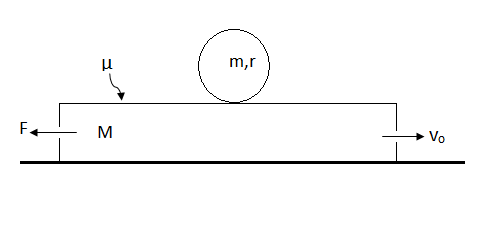# Let it rollA plank of mass $M$ is moving on a smooth horizontal surface with speed $v_{\circ}$. At time $t=0$, a sphere of mass $m$ and radius $r$ is gently placed on it and simultaneously. A constant horizontal force $F$ is applied on the plank in the opposite direction of $v_{\circ}$. Find the time at which sphere starts pure rolling on the plank . The coefficient of friction between the plank and sphere is $\mu$.

$M=4~kg$, $m=2~kg$, $\mu=0.6$, $g=10~m/s^2$,$v_{\circ}=5~ m/s$, $F=40~N$.

×

Problem Loading...

Note Loading...

Set Loading...